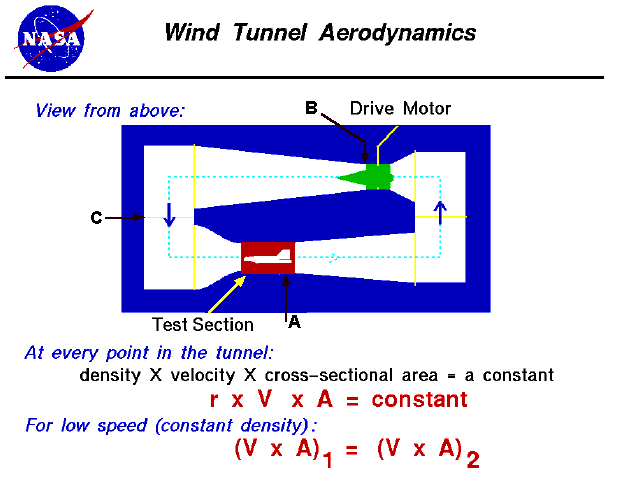+ Text Only Site
+ Non-Flash Version
+ Contact GlennWind Tunnel Aerodynamics Activity If so instructed by your teacher, print out a worksheet page for these problems. Open the slides called Bernoulli's Equation (with text) and Wind Tunnel Aerodynamics (with text) and read the explanations of the relationships between air velocity, pressure, and density in a closed system of varying cross section like a wind tunnel.   Shown below is an image of a typical wind tunnel with three cross sections: A (test section), B (Fan Outlet) and C .The wind tunnel test section (A), as in some operating wind tunnels, can be resized by moving the walls, ceiling and/or floor to give a different cross-sectional area. The other two sections B and C are not adjustable. Give all answers to the nearest hundredth. The measured velocity in the rectangular test section (A) is 10 m/sec. The initial dimensions of the test section are 3 m high and 4 m wide. What is the cross-sectional area (A)? ________________ What is the constant (V x A)? ________________ What cross-sectional area (A) will I need to double the velocity? ________________ What cross-sectional area ( A) will I need to decrease the velocity fourfold? ________________ What would the velocity be if I changed the dimensions to 6 m high and 4 m wide?_____________________________ What would the velocity be if I changed the dimensions to 5 m high and 5 m wide? _____________________________   Assume that the wind tunnel test section (A) is triangular. Our initial velocity is still 10 m/sec. What is the formula for the area of a triangular cross-section? ________________ The triangular test section has a base of 5 m and a height of 4 m. What is the Area (A)? _____________________________ What is the constant (V x A)? _____________________________ What cross-sectional area (A) will I need to double the velocity? _____________________________ Give a base and height measurement which would result in the cross-sectional area in Problem 2d. _____________________________ What would the velocity be if I changed the dimensions to a base of 8 m and a height of 10 m? _____________________________   The fan exit section (B) is circular in shape. The initial velocity is again 10 m/sec. What is the formula for the area of a circular cross section? _____________________________ The cross-sectional area of B is 20 sq. m. What is the radius? _____________________________ What is the constant (V x A)? _____________________________ If I rebuilt this section, what radius would be required to generate a velocity of 50 m/sec.? _____________________________   The cross-sectional areas of Test Section (A), Fan Exit Section (B), and Section C are 20, 60, and 100 m respectively. The velocity at B, the Fan Exit Section, is 50 m/sec. What is the constant (V x A)? _______________________________________ What is the velocity at cross section C? _______________________________________ What is the velocity at Test Section A? _______________________________________

Related Pages:
Standards
Worksheet
Lesson Index
Aerodynamics Index+ Inspector General Hotline + Equal Employment Opportunity Data Posted Pursuant to the No Fear Act + Budgets, Strategic Plans and Accountability Reports + Freedom of Information Act + The President's Management Agenda + NASA Privacy Statement, Disclaimer, and Accessibility CertificationEditor: Tom Benson NASA Official: Tom Benson Last Updated: Fri, May 07 02:42:49 PM EDT 2021 + Contact Glenn